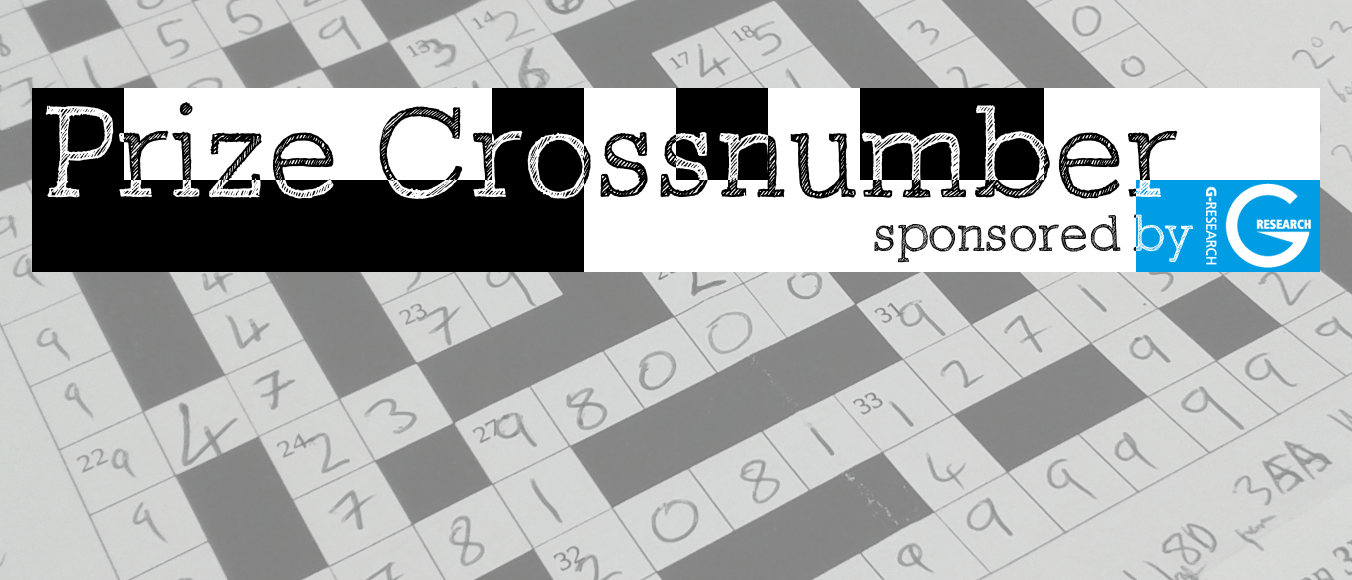# Thoughts on the crossnumber

Did you use meta-logic to solve the crossnumber?The deadline to enter the Chalkdust crossnumber #1 has now passed. The winners will be announced in next week’s blog post. In this blog post, Professor Kevin Buzzard shares some thoughts on the crossnumber.

In the rules we are told that there is a unique solution to the crossnumber. On the face of it this looks like an innocuous comment — a crossnumber for which this wasn’t true would be perhaps a little disappointing (or even unfair). However both existence and uniqueness of a solution to the crossnumber are not immediately obvious, and one has to hence decide what to do with this extra information. One could decide to verify it, by solving the puzzle and checking along the way that there is a unique solution. Alternatively, one could decide to use the information to help solve the puzzle! It is not clear to me if this is “cheating”. Let me give two examples to explain what I mean.The first example is 2 down (“The sum of this number’s digits is equal to 16.“). When I solved the puzzle I knew at some point that the solution to 2 down was of the form $xy5z6$ (with $x,y,z$ denoting unknown digits), and, of the three missing digits, only $x$ formed part of another clue. In particular the only information available about $y$ and $z$ was contained in the clue for 2 down, which was that the sum of the digits was 16, and hence $x+y+z=5$. In particular once we have figured out $x$, we will have to solve the equation $y+z=n$ for $n=5-x$. Existence and uniqueness of the solution to the puzzle then implies that the first digit $x$ must be 5! For if it is more then there will be no solutions, and if it is less than there will be more than one. As it happens I did not use this information, and proved that the first digit was 5 via other means.Here’s another example. Solving the clue for 20 across (“30A more than the largest number which cannot be written as the sum of distinct fourth powers.“) involves knowing the largest positive integer $N$ that is not the sum of distinct 4th powers. Evaluating this number by hand from first principles is a rather tedious computation. However, once one knows 20 across begins with a 5, if we assume existence of a solution to the puzzle then N must be at most 6 million, so all we have to do is to figure out which numbers less than 6 million are not the sums of distinct 4th powers, and using a computer this can be done in well under a minute (exercise: come up with an algorithm!). Once we have evaluated this set, $N$ must be the largest number in it. This was the method I used, and this was the only time I used such “meta-analysis” — hence ultimately my initial solution to the crossnumber assumed existence but proved uniqueness. Later on I proved (using a computer and an inductive argument) that $N$ was indeed 5134240, so ultimately I did verify both existence and uniqueness of the solution; however I guess that logically this was not necessary.Kevin is a professor of pure mathematics at Imperial College London. The Xena Project meets to discuss Lean every Thursday evening during term time in the computer room of the maths department at Imperial College; bring a laptop. He is currently writing a book for undergraduate mathematicians about how to learn Lean.
@xenaproject    xenaproject.wordpress.com    + More articles by Kevin